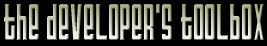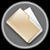This section of the archives stores flipcode's complete Developer Toolbox collection, featuring a variety of mini-articles and source code contributions from our readers.Calculating Vertex Normals for Height Maps   Submitted byI had the problem that for surfaces defined by a heightmap like terrain or water calculating the vertex normals the normal way, by averaging all adjacent surface normals after the heightmap is triangulated, seemed to be slow (especially for a big heightmap and if you do it often, like every frame for water).

Now what I do is calculate the vertex normal only based on the height map (similar to converting a heightmap to a normal map I guess). For every vertex normal I take 4 samples (left, right, top bottom of the current heightmap pixel) this gives me the average slopes in x and z direction. This results in two "2D" vertex normals (one in x, one in z direction). Now I only have to find a 3D vector, that projected to the x=0, z=0 planes gives the same slopes as the "2D" normals and normalize it. Well, maybe it's best explained by looking at the code snippet below. The method should be considerably faster than doing all those crossproducts.

(The normals calculated this way are independent of the later triangulation of the heightmap, so they are not the same you'd get by averaging the surface normals, but this is not noticeable in the result.)

 ```// unsigned char h(x, y) returns the height map value at x, y. // the map is of size width*height // Vector3 normal[width*height] will contain the calculated normals. // // The height map has x, y axes with (0, 0) being the top left corner of the map. // The resulting mesh is assumed to be in a left hand system with x right, z into the screen // and y up (i.e. as in DirectX). // // yScale denotes the scale of mapping heights to final y values in model space // (i.e. a height difference of 1 in the height map results in a height difference // of yScale in the vertex coordinate). // xzScale denotes the same for the x, z axes. If you have different scale factors // for x, z then the formula becomes // normal[y*width+x].set(-sx*yScale, 2*xScale, xScalesy*xScale*yScale/zScale); for (unsigned int y = 0; y

The zip file viewer built into the Developer Toolbox made use of the zlib library, as well as the zlibdll source additions.